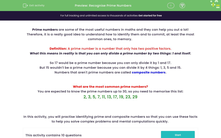# Recognise Prime Numbers

In this worksheet, students will practise identifying prime and composite numbers, so that these facts can be used to solve complex problems and mental computations quickly.Key stage:  KS 4

Year:  GCSE

GCSE Subjects:   Maths

GCSE Boards:   Pearson Edexcel, OCR, Eduqas, AQA,

Curriculum topic:   Number, Number Operations and Integers

Curriculum subtopic:   Structure and Calculation Whole Number Theory

Popular topics:   Numbers worksheets, Prime Numbers worksheets, Factors worksheets

Difficulty level:#### Worksheet Overview

Prime numbers are some of the most useful numbers in maths and they can help you out a lot!

Therefore, it is a really good idea to understand how to identify them and to commit, at least the most common ones, to memory.

Definition: A prime number is a number that only has two positive factors.

What this means in reality is that you can only divide a prime number by two things: 1 and itself.

So 17 would be a prime number because you can only divide it by 1 and 17.

But 15 wouldn't be a prime number because you can divide it by 4 things: 1, 3, 5 and 15.

Numbers that aren't prime numbers are called composite numbers.

What are the most common prime numbers?

You are expected to know the prime numbers up to 30, so you need to memorise this list:

2, 3, 5, 7, 11, 13, 17, 19, 23, 29

In this activity, you will practise identifying prime and composite numbers so that you can use these facts to help you solve complex problems and mental computations quickly.

### What is EdPlace?

We're your National Curriculum aligned online education content provider helping each child succeed in English, maths and science from year 1 to GCSE. With an EdPlace account you’ll be able to track and measure progress, helping each child achieve their best. We build confidence and attainment by personalising each child’s learning at a level that suits them.

Get started#### Popular Maths topics

••••